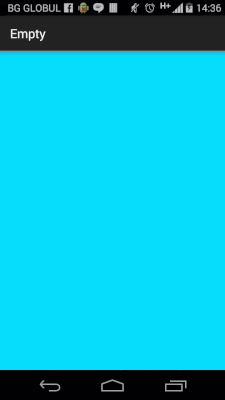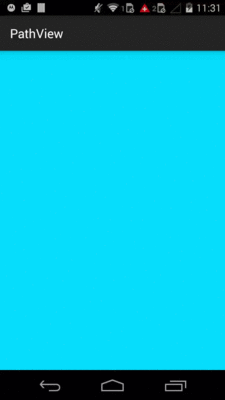# 安卓自定义View进阶-PathMeasure

2022-12-08 21:55:46 michael007js 62

PS：不要问我为什么不讲 PathEffect，因为这个方法在后面的Paint系列中。## Path & PathMeasure

### 公共方法

PathMeasure的方法也不多，接下来我们就逐一的讲解一下。

### 1.构造函数

`PathMeasure ()`

`PathMeasure (Path path, boolean forceClosed)`

1. 不论 forceClosed 设置为何种状态(true 或者 false)， 都不会影响原有Path的状态，即 Path 与 PathMeasure 关联之后，之前的的 Path 不会有任何改变。

1. forceClosed 的设置状态可能会影响测量结果，如果 Path 未闭合但在与 PathMeasure 关联的时候设置 forceClosed 为 true 时，测量结果可能会比 Path 实际长度稍长一点，获取到到是该 Path 闭合时的状态。

`canvas.translate(mViewWidth/2,mViewHeight/2);Path path = new Path();path.lineTo(0,200);path.lineTo(200,200);path.lineTo(200,0);PathMeasure measure1 = new PathMeasure(path,false);PathMeasure measure2 = new PathMeasure(path,true);Log.e("TAG", "forceClosed=false---->"+measure1.getLength());Log.e("TAG", "forceClosed=true----->"+measure2.getLength());canvas.drawPath(path,mDeafultPaint);`

log如下:

`com.gcssloop.canvas E/TAG: forceClosed=false---->600.0com.gcssloop.canvas E/TAG: forceClosed=true----->800.0`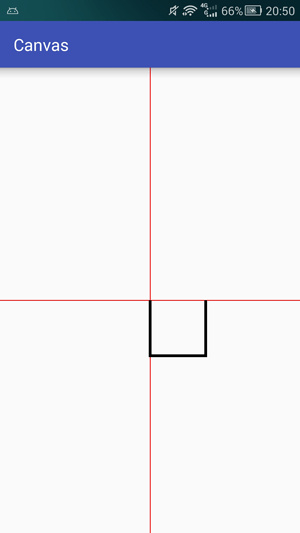• 1.我们将 Path 与两个的 PathMeasure 进行关联，并给 forceClosed 设置了不同的状态，之后绘制再绘制出来的 Path 没有任何变化，所以与 Path 与 PathMeasure进行关联并不会影响 Path 状态。

• 2.我们可以看到，设置 forceClosed 为 true 的方法比设置为 false 的方法测量出来的长度要长一点，这是由于 Path 没有闭合的缘故，多出来的距离正是 Path 最后一个点与最开始一个点之间点距离。forceClosed 为 false 测量的是当前 Path 状态的长度， forceClosed 为 true，则不论Path是否闭合测量的都是 Path 的闭合长度。

### 2.setPath、 isClosed 和 getLength

setPath 是 PathMeasure 与 Path 关联的重要方法，效果和 构造函数 中两个参数的作用是一样的。

isClosed 用于判断 Path 是否闭合，但是如果你在关联 Path 的时候设置 forceClosed 为 true 的话，这个方法的返回值则一定为true。

getLength 用于获取 Path 的总长度，在之前的测试中已经用过了。

### 3.getSegment

getSegment 用于获取Path的一个片段，方法如下：

`boolean getSegment (float startD, float stopD, Path dst, boolean startWithMoveTo)`

• 如果 startD、stopD 的数值不在取值范围 [0, getLength] 内，或者 startD == stopD 则返回值为 false，不会改变 dst 内容。

• 如果在安卓4.4或者之前的版本，在默认开启硬件加速的情况下，更改 dst 的内容后可能绘制会出现问题，请关闭硬件加速或者给 dst 添加一个单个操作，例如: dst.rLineTo(0, 0)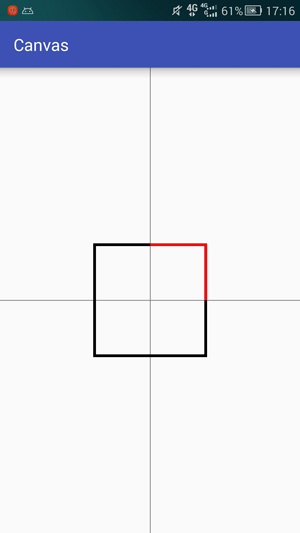`canvas.translate(mViewWidth / 2, mViewHeight / 2);        // 平移坐标系Path path = new Path();                                     // 创建Path并添加了一个矩形path.addRect(-200, -200, 200, 200, Path.Direction.CW);Path dst = new Path();                                    // 创建用于存储截取后内容的 PathPathMeasure measure = new PathMeasure(path, false);         // 将 Path 与 PathMeasure 关联// 截取一部分存入dst中，并使用 moveTo 保持截取得到的 Path 第一个点的位置不变measure.getSegment(200, 600, dst, true);                    canvas.drawPath(dst, mDeafultPaint);                      // 绘制 dst`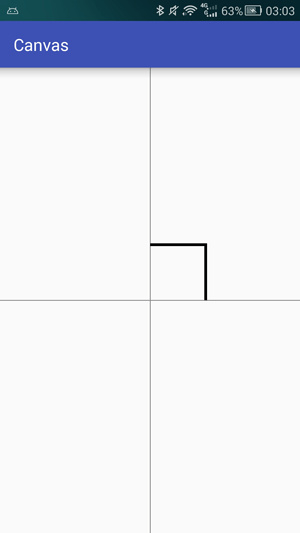`canvas.translate(mViewWidth / 2, mViewHeight / 2);        // 平移坐标系Path path = new Path();                                     // 创建Path并添加了一个矩形path.addRect(-200, -200, 200, 200, Path.Direction.CW);Path dst = new Path();                                    // 创建用于存储截取后内容的 Pathdst.lineTo(-300, -300);                                     // <--- 在 dst 中添加一条线段PathMeasure measure = new PathMeasure(path, false);         // 将 Path 与 PathMeasure 关联measure.getSegment(200, 600, dst, true);                   // 截取一部分 并使用 moveTo 保持截取得到的 Path 第一个点的位置不变canvas.drawPath(dst, mDeafultPaint);                      // 绘制 Path`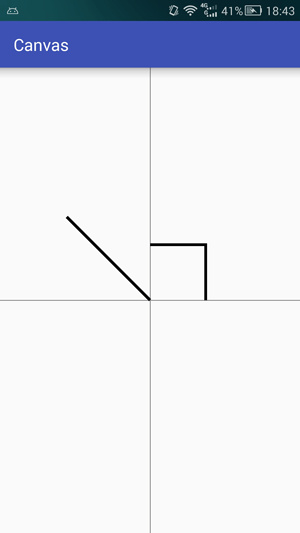`canvas.translate(mViewWidth / 2, mViewHeight / 2);        // 平移坐标系Path path = new Path();                                     // 创建Path并添加了一个矩形path.addRect(-200, -200, 200, 200, Path.Direction.CW);Path dst = new Path();                                    // 创建用于存储截取后内容的 Pathdst.lineTo(-300, -300);                                     // 在 dst 中添加一条线段PathMeasure measure = new PathMeasure(path, false);         // 将 Path 与 PathMeasure 关联measure.getSegment(200, 600, dst, false);                   // <--- 截取一部分 不使用 startMoveTo, 保持 dst 的连续性canvas.drawPath(dst, mDeafultPaint);                      // 绘制 Path`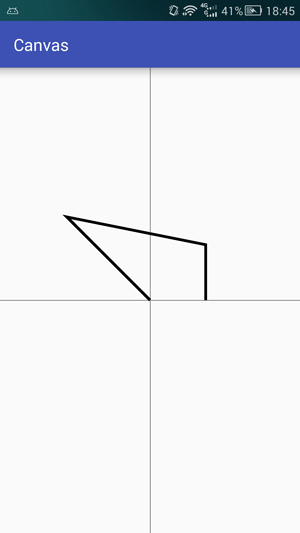### 4.nextContour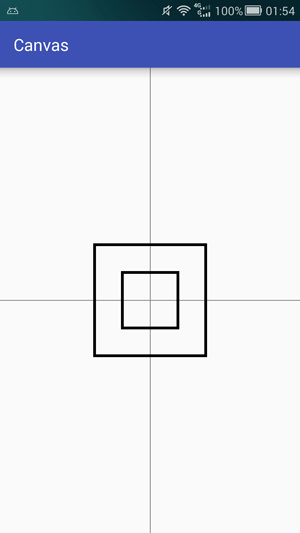`canvas.translate(mViewWidth / 2, mViewHeight / 2);    // 平移坐标系Path path = new Path();path.addRect(-100, -100, 100, 100, Path.Direction.CW);// 添加小矩形path.addRect(-200, -200, 200, 200, Path.Direction.CW);// 添加大矩形canvas.drawPath(path,mDeafultPaint);                  // 绘制 PathPathMeasure measure = new PathMeasure(path, false);     // 将Path与PathMeasure关联float len1 = measure.getLength();                       // 获得第一条路径的长度measure.nextContour();                                // 跳转到下一条路径float len2 = measure.getLength();                       // 获得第二条路径的长度Log.i("LEN","len1="+len1);                            // 输出两条路径的长度Log.i("LEN","len2="+len2);`

log输出结果:

`com.gcssloop.canvas I/LEN: len1=800.0com.gcssloop.canvas I/LEN: len2=1600.0`

• 1.曲线的顺序与 Path 中添加的顺序有关。

• 2.getLength 获取到到是当前一条曲线分长度，而不是整个 Path 的长度。

• 3.getLength 等方法是针对当前的曲线(其它方法请自行验证)。

#### 5.getPosTan

`boolean getPosTan (float distance, float[] pos, float[] tan)`

`tan` 是用来判断 Path 上趋势的，即在这个位置上曲线的走向，请看下图示例，注意箭头的方向:`private float currentValue = 0;     // 用于纪录当前的位置,取值范围[0,1]映射Path的整个长度private float[] pos;              // 当前点的实际位置private float[] tan;              // 当前点的tangent值,用于计算图片所需旋转的角度private Bitmap mBitmap;             // 箭头图片private Matrix mMatrix;             // 矩阵,用于对图片进行一些操作`

`private void init(Context context) {  pos = new float;  tan = new float;  BitmapFactory.Options options = new BitmapFactory.Options();  options.inSampleSize = 2;       // 缩放图片  mBitmap = BitmapFactory.decodeResource(context.getResources(), R.drawable.arrow, options);  mMatrix = new Matrix();}`

`canvas.translate(mViewWidth / 2, mViewHeight / 2);    // 平移坐标系Path path = new Path();                                 // 创建 Pathpath.addCircle(0, 0, 200, Path.Direction.CW);           // 添加一个圆形PathMeasure measure = new PathMeasure(path, false);     // 创建 PathMeasurecurrentValue += 0.005;                                // 计算当前的位置在总长度上的比例[0,1]if (currentValue >= 1) {currentValue = 0;}measure.getPosTan(measure.getLength() * currentValue, pos, tan);      // 获取当前位置的坐标以及趋势mMatrix.reset();                                                      // 重置Matrixfloat degrees = (float) (Math.atan2(tan, tan) * 180.0 / Math.PI); // 计算图片旋转角度mMatrix.postRotate(degrees, mBitmap.getWidth() / 2, mBitmap.getHeight() / 2);   // 旋转图片mMatrix.postTranslate(pos - mBitmap.getWidth() / 2, pos - mBitmap.getHeight() / 2);   // 将图片绘制中心调整到与当前点重合canvas.drawPath(path, mDeafultPaint);                                   // 绘制 Pathcanvas.drawBitmap(mBitmap, mMatrix, mDeafultPaint);                     // 绘制箭头invalidate();                                                           // 重绘页面`

• 1.通过 `tan` 得值计算出图片旋转的角度，tan 是 tangent 的缩写，即中学中常见的正切， 其中tan是邻边边长，tan是对边边长，而Math中 `atan2` 方法是根据正切是数值计算出该角度的大小,得到的单位是弧度(取值范围是 -pi 到 pi)，所以上面又将弧度转为了角度。

• 2.通过 `Matrix` 来设置图片对旋转角度和位移，这里使用的方法与前面讲解过对 canvas操作 有些类似，对于 `Matrix` 会在后面专一进行讲解，敬请期待。

• 3.页面刷新，页面刷新此处是在 onDraw 里面调用了 invalidate 方法来保持界面不断刷新，但并不提倡这么做，正确对做法应该是使用 线程 或者 ValueAnimator 来控制界面的刷新，关于控制页面刷新这一部分会在后续的 动画部分 详细讲解，同样敬请期待。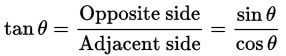tan = cos = 邻边(单位圆x坐标) tan = sin = 对边(单位圆y坐标)

`sin／cos`理解: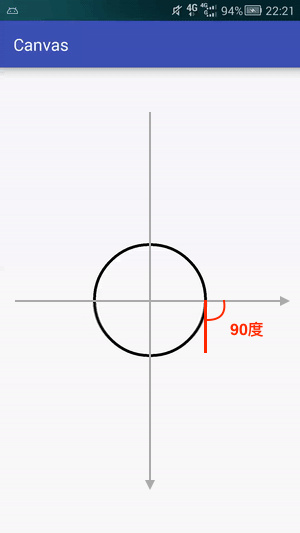`对边／邻边` 理解(单位圆上坐标):tan30 = 对边／邻边 = AB／OA = B点y坐标／B点x坐标

PS: 使用 Math.atan2(tan, tan) 将 `tan` 转化为角(单位为弧度)的时候要注意参数顺序。

### 6.getMatrix

`boolean getMatrix (float distance, Matrix matrix, int flags)`

`measure.getMatrix(distance, matrix, PathMeasure.TANGENT_MATRIX_FLAG | PathMeasure.POSITION_MATRIX_FLAG);`

`Path path = new Path();                                 // 创建 Pathpath.addCircle(0, 0, 200, Path.Direction.CW);           // 添加一个圆形PathMeasure measure = new PathMeasure(path, false);     // 创建 PathMeasurecurrentValue += 0.005;                                // 计算当前的位置在总长度上的比例[0,1]if (currentValue >= 1) {  currentValue = 0;}// 获取当前位置的坐标以及趋势的矩阵measure.getMatrix(measure.getLength() * currentValue, mMatrix, PathMeasure.TANGENT_MATRIX_FLAG | PathMeasure.POSITION_MATRIX_FLAG);mMatrix.preTranslate(-mBitmap.getWidth() / 2, -mBitmap.getHeight() / 2);   // <-- 将图片绘制中心调整到与当前点重合(注意:此处是前乘pre)canvas.drawPath(path, mDeafultPaint);                                   // 绘制 Pathcanvas.drawBitmap(mBitmap, mMatrix, mDeafultPaint);                     // 绘制箭头invalidate();                                                           // 重绘页面`

• 1.对 `matrix` 的操作必须要在 `getMatrix` 之后进行，否则会被 `getMatrix` 重置而导致无效。

• 2.矩阵对旋转角度默认为图片的左上角，我们此处需要使用 `preTranslate` 调整为图片中心。

• 3.pre(矩阵前乘) 与 post(矩阵后乘) 的区别，此处请等待后续的文章或者自行搜索。

## Path & SVG

SVG 是一种矢量图，内部用的是 xml 格式化存储方式存储这操作和数据，你完全可以将 SVG 看作是 Path 的各项操作简化书写后的存储格式。

Path 和 SVG 结合通常能诞生出一些奇妙的东西，如下: